<Github - qqwweee/keras-yolo3>

## 1. 目标检测基础

### 1.2. 精确率与召回率

Predicted NegativePredicted Positive
Negative CasesTN: 9760FP: 140
Positive CasesFN: 40TP: 60

• True positives : 正样本被正确识别为正样本，飞机的图片被正确的识别成了飞机.
• True negatives: 负样本被正确识别为负样本，大雁的图片没有被识别出来，系统正确地认为它们是大雁.
• False positives: 假的正样本，即负样本被错误识别为正样本，大雁的图片被错误地识别成了飞机.
• False negatives: 假的负样本，即正样本被错误识别为负样本，飞机的图片没有被识别出来，系统错误地认为它们是大雁.
• Precision 其实就是在识别出来的图片中，True positives所占的比率. 也就是本假设中，所有被识别出来的飞机中，真正的飞机所占的比例.

$$Precision = \frac{TP}{TP + FP}$$

• Recall 是测试集中所有正样本样例中，被正确识别为正样本的比例. 也就是本假设中，被正确识别出来的飞机个数与测试集中所有真实飞机的个数的比值.

$$Recall = \frac{TP}{TP + FN}$$

• Precision-recall 曲线：改变识别阈值，使得系统依次能够识别前K张图片，阈值的变化同时会导致Precision与Recall值发生变化，从而得到曲线.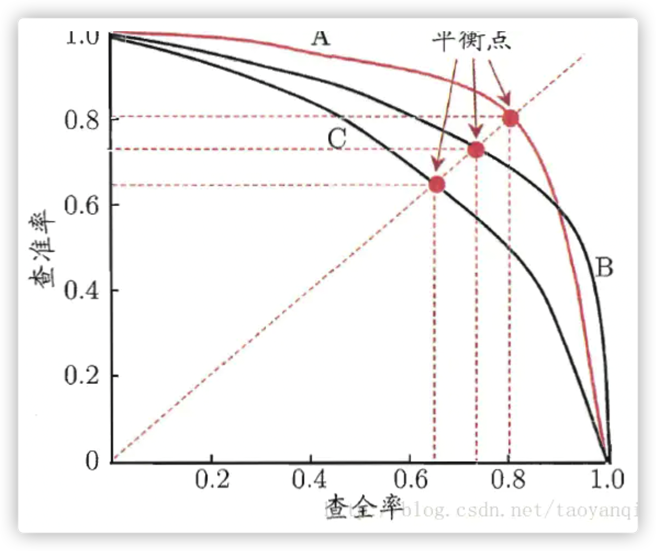### 1.3. 平均精度AP 与多类别平均精度mAP

AP 就是Precision-recall 曲线下面的面积，通常来说一个越好的分类器，AP值越高.

mAP 是多个类别AP的平均值. 这个mean的意思是对每个类的AP再求平均，得到的就是mAP的值，mAP的大小一定在[0,1]区间，越大越好. 该指标是目标检测算法中最重要的一个.

### 1.4. IoU

IoU这一值，可以理解为系统预测出来的框与原来图片中标记的框的重合程度.

IoU正是表达这种bounding box和groundtruth的差异的指标：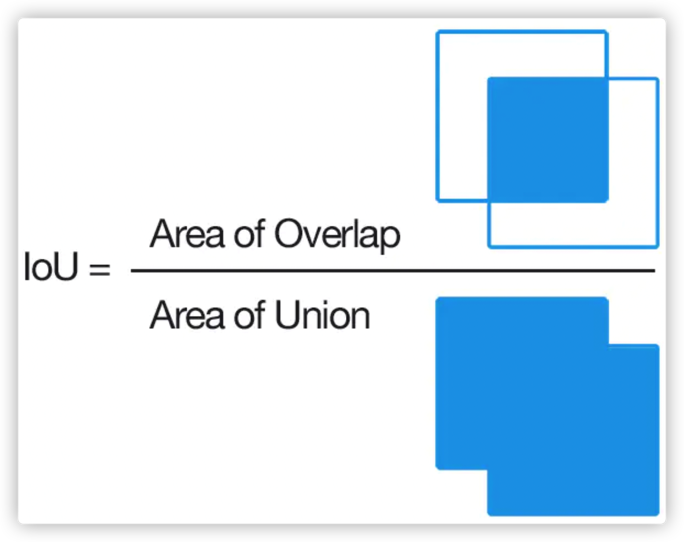### 1.5. 非极大值抑制(NMS)

Non-Maximum Suppression 就是需要根据 score 矩阵和 region 的坐标信息，从中找到置信度比较高的bounding box. 对于有重叠在一起的预测框，只保留得分最高的那个.

 - NMS计算出每一个bounding box的面积，然后根据score进行排序，把score最大的bounding box作为队列中首个要比较的对象.

 - 计算其余bounding box与当前最大score与box的IoU，去除IoU大于设定的阈值的bounding box，保留小的IoU得预测框.

 - 然后重复上面的过程，直至候选bounding box为空.

### 1.7. One Stage & Two Stage

YOLOV3，SSD，RetinaNet都属于one stage类型的网络，这类网络的特点是训练和识别速度快，但是精度欠佳.
Faster RCNN和Mask RCNN属于two stage类型的网络，相比于one stage，识别精度上有所提升，但是训练和识别速度比不上one stage类型的网络.

## 2. YOLOV3

### 2.1. YOLOV3 网络结构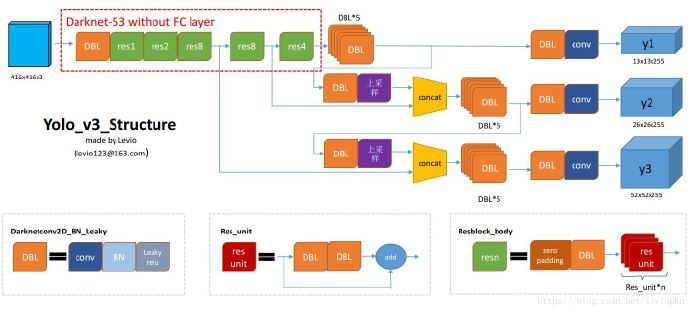DBL: 卷积层Conv + 批标准化层BN + Leaky Relu.

res(n): n 代表这个 res_block 内含有多少个 res_unit，这点借鉴了ResNet的残差结构，使用这种结构的目的是为了加深网络深度

concat: 将DarkNet中的某一层与之前的某层的上采样.

YOLOV3 流程如下:

 - 调整输入图像的大小为416 × 416(32的倍数)

 - 图像向前传播的过程中经过一个 1 个DBL层和 5 个res_block，每经过一个res_block，图像的size 都要减半，此时图像的 size为416 / 32(2的5次方) = 13 * 13.

 - 下图是一张DarkNet-53的结构图，然而 YOLOV3 经过前面的 res_block 后不是继续采用接下来的 Avgpool 平均池化层，Connected，全连接层，而是继续经过5个DBL层.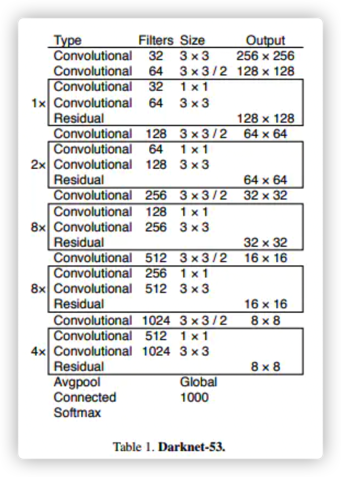- 接下来有两步操作:

(4.1) - 经过一个 DBL层和卷积层 conv 得到输出y1(13 13 255)，这里的255是9 / 3 * (4 + 1 + 80). 对这几个数字的说明如下:

• 9 是 anchors的数量，这里的anchor的数量是通过聚类得到的.
• 除以3是因为最终的输出的特征图有3个 scale (13，26，52)，13 * 13 对应的是9个anchors里top3 大的锚框.
• 4代表的每个锚框中心的横坐标x，纵坐标y,宽度w,高度h.
• 1和80分别表示背景和80目标种类的概率

(4.2) - 通过一个DBL和一个上采样层和 res_block4 的输出连接起来，然后经过5个DBL层.

 - 步骤(4.2)的结果也有两步操作:
(5.1) - 经过一个 DBL 层和卷积层 conv 得到输出y2(26 26 255)，26是因为res_block4的输出特征图大小为26，而步骤(4.1)的输入经过上采样的操作后特征图大小也从13变成了26.
(5.2) - 通过一个DBL和一个上采样层和res_block3的输出连接起来，然后经过5个DBL层.

 - 将步骤(5.2) 的结果经过一个 DBL 层和一个上采样层与 res_block3 的输出连接起来，再经过6(5+1)个 DBL 层和一个卷积层conv得到y3(52 52 255).

### 2.2. YOLOV3 Loss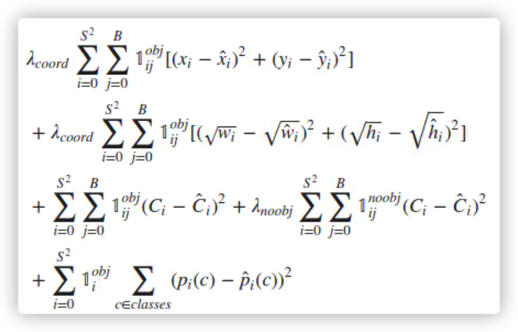#### 2.2.1. 坐标误差

λcoord 在 YOLOV1 中默认为 5，因为目标的定位是最重要的部分，所以给定位损失一个比较高的权重. 但是在看代码的时候发现这个值变成了 $2 - w \cdot h$ (w, h 都归一化到[0,1])，应该是降低了一些权重，同时将物体的大小考虑进去，从公式中可以发现小的物体拥有更高的权重，因为对于小物体，几个像素的误差带来的影响是高于大的物体.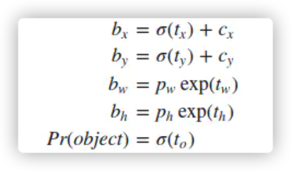$c_x, c_y$ 是位移偏差offset，$\sigma ()$ 函数为logistic函数，将坐标归一化到[0，1].

raw_true_wh = K.log(y_true[l][..., 2:4] / anchors[anchor_mask[l]] * input_shape[::-1])
raw_true_wh = K.switch(object_mask, raw_true_wh, K.zeros_like(raw_true_wh)) # avoid log(0)=-inf
#......此处省略中间的一些代码，直接看w和h的误差计算
wh_loss = object_mask * box_loss_scale * 0.5 * K.square(raw_true_wh-raw_pred[...,2:4])

YOLOV3 跟 YOLOV1 对于宽高的损失计算也有些区别，YOLOV1是 $(\sqrt {w} - \sqrt{w'} )^2$；YOLOV3是$(log(w) - log(w')))^2$，不过效果是一样的，都是提高对于小目标的预测敏感度. 举个简单的例子，同样是10个像素的误差，一个大的目标真实的宽为100，预测出来为110；而一个小的目标真实宽度为10，预测出来是20，让我们来通过这个公式计算一下误差:
0.5 * (log(110) - log(100))^2 = 0.00085667719
0.5 * (log(20) - log(10))^2 = 0.04530952914

#### 2.2.2. IOU 误差

λnoobj在源码中没有找到这个参数，YOLOV1是设置来减少正反例分布不均匀带来的误差的，作者为什么要这么做，作者暂还没找到原因，猜测是对于这种分布不均衡问题我们没有必要去干预它，顺其自然就好.

#### 2.2.3. 分类误差

class_loss = object_mask * K.binary_crossentropy(
true_class_probs,
raw_pred[...,5:],
from_logits=True)

### 2.3. YOLOV3 检测示例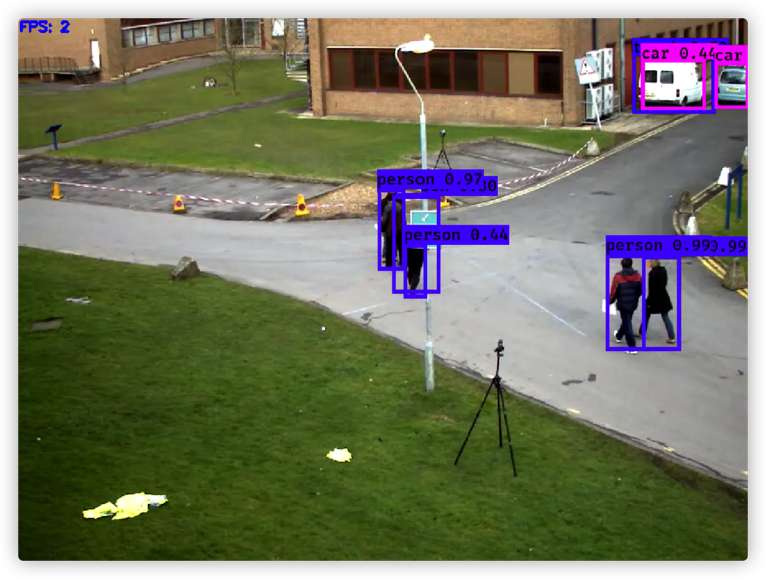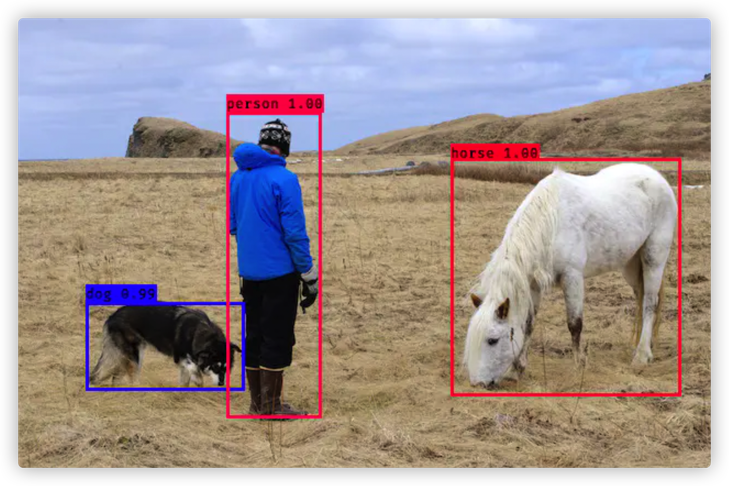### 2.4. YOLOV3 检测流程代码

1. 初始化

self.__dict__.update(self._defaults) # set up default values
self.__dict__.update(kwargs) # and update with user overrides
self.class_names = self._get_class()
self.anchors = self._get_anchors()
self.sess = K.get_session()
self.boxes, self.scores, self.classes = self.generate()

    def generate(self):
model_path = os.path.expanduser(self.model_path)
assert model_path.endswith('.h5'), 'Keras model or weights must be a .h5 file.'

num_anchors = len(self.anchors)
num_classes = len(self.class_names)
is_tiny_version = num_anchors==6 # default setting
try:
except:
self.yolo_model = tiny_yolo_body(
Input(shape=(None,None,3)),
num_anchors//2,
num_classes)
if is_tiny_version else
yolo_body(Input(shape=(None,None,3)),
num_anchors//3,
num_classes)
# make sure model, anchors and classes match
else:
assert self.yolo_model.layers[-1].output_shape[-1] == \
num_anchors/len(self.yolo_model.output) * (num_classes + 5), \
'Mismatch between model and given anchor and class sizes'

print('{} model, anchors, and classes loaded.'.format(model_path))

self.input_image_shape = K.placeholder(shape=(2, ))
if self.gpu_num>=2:
self.yolo_model = multi_gpu_model(self.yolo_model, gpus=self.gpu_num)
boxes, scores, classes = yolo_eval(self.yolo_model.output,
self.anchors,
len(self.class_names),
self.input_image_shape,
score_threshold=self.score,
iou_threshold=self.iou)
return boxes, scores, classes

def yolo_correct_boxes(box_xy, box_wh, input_shape, image_shape):
'''
Get corrected boxes
'''
box_yx = box_xy[..., ::-1]
box_hw = box_wh[..., ::-1]
input_shape = K.cast(input_shape, K.dtype(box_yx))
image_shape = K.cast(image_shape, K.dtype(box_yx))
new_shape = K.round(image_shape * K.min(input_shape/image_shape))
offset = (input_shape-new_shape)/2./input_shape
scale = input_shape/new_shape
box_yx = (box_yx - offset) * scale
box_hw *= scale

box_mins = box_yx - (box_hw / 2.)
box_maxes = box_yx + (box_hw / 2.)
boxes =  K.concatenate([
box_mins[..., 0:1],  # y_min
box_mins[..., 1:2],  # x_min
box_maxes[..., 0:1],  # y_max
box_maxes[..., 1:2]  # x_max
])

# Scale boxes back to original image shape.
boxes *= K.concatenate([image_shape, image_shape])
return boxes

def yolo_boxes_and_scores(feats, anchors, num_classes, input_shape, image_shape):
'''
Process Conv layer output
'''
box_xy, box_wh, box_confidence, box_class_probs = yolo_head(
feats,anchors, num_classes, input_shape)
boxes = yolo_correct_boxes(box_xy, box_wh, input_shape, image_shape)
boxes = K.reshape(boxes, [-1, 4])
box_scores = box_confidence * box_class_probs
box_scores = K.reshape(box_scores, [-1, num_classes])
return boxes, box_scores

def yolo_eval(yolo_outputs,
anchors,
num_classes,
image_shape,
max_boxes=20,
score_threshold=.6,
iou_threshold=.5):
"""
Evaluate YOLO model on given input and return filtered boxes.
"""
num_layers = len(yolo_outputs)
anchor_mask = [[6,7,8], [3,4,5], [0,1,2]] if num_layers==3 else [[3,4,5], [1,2,3]] # default setting
input_shape = K.shape(yolo_outputs)[1:3] * 32
boxes = []
box_scores = []
for l in range(num_layers):
_boxes, _box_scores = yolo_boxes_and_scores(
yolo_outputs[l],
num_classes,
input_shape,
image_shape)
boxes.append(_boxes)
box_scores.append(_box_scores)
boxes = K.concatenate(boxes, axis=0)
box_scores = K.concatenate(box_scores, axis=0)

mask = box_scores >= score_threshold
max_boxes_tensor = K.constant(max_boxes, dtype='int32')

boxes_ = []
scores_ = []
classes_ = []
for c in range(num_classes):
# TODO: use keras backend instead of tf.
nms_index = tf.image.non_max_suppression(
class_boxes,
class_box_scores,
max_boxes_tensor,
iou_threshold=iou_threshold)
class_boxes = K.gather(class_boxes, nms_index)
class_box_scores = K.gather(class_box_scores, nms_index)
classes = K.ones_like(class_box_scores, 'int32') * c
boxes_.append(class_boxes)
scores_.append(class_box_scores)
classes_.append(classes)
boxes_ = K.concatenate(boxes_, axis=0)
scores_ = K.concatenate(scores_, axis=0)
classes_ = K.concatenate(classes_, axis=0)

return boxes_, scores_, classes_

self.boxes, self.scores, self.classes = self.generate()

### 2.5. YOLOV3 图片预处理代码

def letterbox_image(image, size):
'''
resize image with unchanged aspect ratio using padding.
'''
iw, ih = image.size
w, h = size
scale = min(w/iw, h/ih)
nw = int(iw*scale)
nh = int(ih*scale)

image = image.resize((nw,nh), Image.BICUBIC)
new_image = Image.new('RGB', size, (128,128,128))
new_image.paste(image, ((w-nw)//2, (h-nh)//2))

return new_image
if self.model_image_size != (None, None):
assert self.model_image_size%32 == 0, 'Multiples of 32 required'
assert self.model_image_size%32 == 0, 'Multiples of 32 required'
boxed_image = letterbox_image(
image, tuple(reversed(self.model_image_size)))
else:
new_image_size = (image.width - (image.width % 32),
image.height - (image.height % 32))
boxed_image = letterbox_image(image, new_image_size) #
image_data = np.array(boxed_image, dtype='float32')

image_data /= 255.
image_data = np.expand_dims(image_data, 0)  # Add batch dimension.

### 2.6. YOLOV3 前向计算代码

out_boxes, out_scores, out_classes = self.sess.run(
[self.boxes, self.scores, self.classes],
feed_dict={self.yolo_model.input: image_data,
self.input_image_shape: [image.size, image.size],
K.learning_phase(): 0}
)

print('Found {} boxes for {}'.format(len(out_boxes), 'img'))

## 3. 目标检测识别精度与速度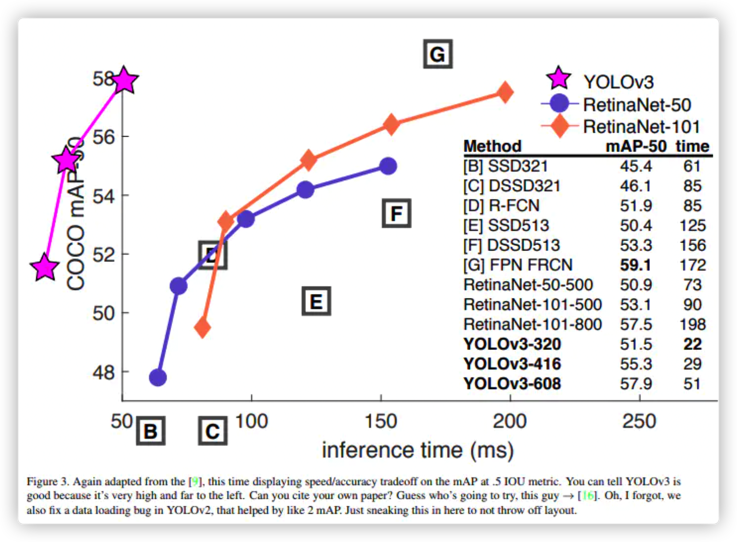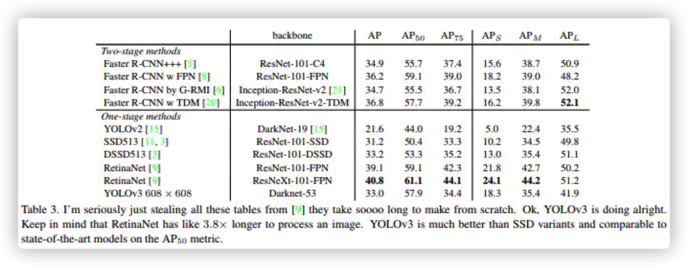Last modification：February 2nd, 2021 at 11:42 am